• knn机器学习算法Goal: To classify a query point (with 2 features) using training data of 2 classes using KNN. 目标：使用KNN使用2类的训练数据对查询点(具有2个要素)进行分类。 K最近邻居(KNN) (K- Nearest...
knn机器学习算法Goal: To classify a query point (with 2 features) using training data of 2 classes using KNN.
目标：使用KNN使用2类的训练数据对查询点(具有2个要素)进行分类。
K最近邻居(KNN) (K- Nearest Neighbor (KNN))
KNN is a basic machine learning algorithm that can be used for both classifications as well as regression problems but has limited uses as a regression problem. So, we would discuss classification problems only.
KNN是一种基本的机器学习算法，可用于分类和回归问题，但作为回归问题用途有限。 因此，我们仅讨论分类问题。
It involves finding the distance of a query point with the training points in the training datasets. Sorting the distances and picking k points with the least distance. Then check which class these k points belong to and the class with maximum appearance is the predicted class.
它涉及在训练数据集中找到查询点与训练点之间的距离。 排序距离并选择距离最小的k个点。 然后检查这k个点属于哪个类别，并且外观最大的类别是预测的类别。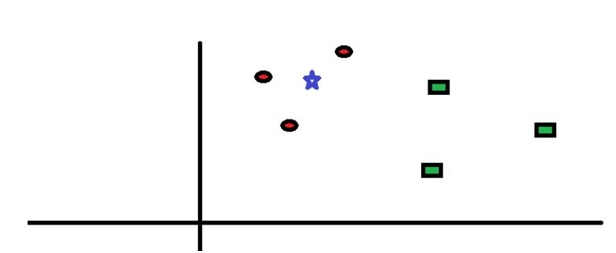Red and green are two classes here, and we have to predict the class of star point. So, from the image, it is clear that the points of the red class are much closer than points of green class so the class prediction will be red for this point.
红色和绿色是这里的两个类别，我们必须预测星点的类别。 因此，从图像中可以明显看出，红色类别的点比绿色类别的点近得多，因此该类别的预测将是红色。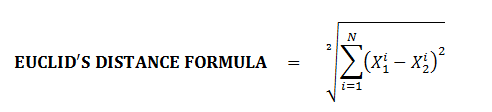We will generally work on the matrix, and make use of "numpy" libraries to evaluate this Euclid’s distance.
通常，我们将在矩阵上工作，并使用“ numpy”库来评估该Euclid的距离。
Algorithm:
算法：
STEP 1: Take the distance of a query point or a query reading from all the training points in the training dataset. 步骤1：从训练数据集中的所有训练点获取查询点或查询读数的距离。 STEP 2: Sort the distance in increasing order and pick the k points with the least distance. 步骤2：按递增顺序对距离进行排序，并选择距离最小的k个点。 STEP 3: Check the majority of class in these k points. 步骤3：在这k点中检查大部分班级。 STEP 4: Class with the maximum majority is the predicted class of the point. 步骤4：具有最大多数的类别是该点的预测类别。 Note: In the code, we have taken only two features for a better explanation but the code works for N features also just you have to generate training data of n features and a query point of n features. Further, I have used numpy to generate two feature data.
注：在代码中，我们采取了只有两个功能，一个更好的解释，但该代码适用于N个特征也只是你要生成的n个特征和n个特征查询点的训练数据。 此外，我使用numpy生成了两个特征数据。
Python Code
Python代码
import numpy as np

def distance(v1, v2):
# Eucledian
return np.sqrt(((v1-v2)**2).sum())

def knn(train, test, k=5):
dist = []

for i in range(train.shape):
# Get the vector and label
ix = train[i, :-1]
iy = train[i, -1]
# Compute the distance from test point
d = distance(test, ix)
dist.append([d, iy])
# Sort based on distance and get top k
dk = sorted(dist, key=lambda x: x)[:k]
# Retrieve only the labels
labels = np.array(dk)[:, -1]

# Get frequencies of each label
output = np.unique(labels, return_counts=True)
# Find max frequency and corresponding label
index = np.argmax(output)
return output[index]

# monkey_data && chimp data
# Data has 2 features
monkey_data = np.random.multivariate_normal([1.0,2.0],[[1.5,0.5],[0.5,1]],1000)
chimp_data = np.random.multivariate_normal([4.0,4.0],[[1,0],[0,1.8]],1000)

data = np.zeros((2000,3))
data[:1000,:-1] = monkey_data
data[1000:,:-1] = chimp_data
data[1000:,-1] = 1

label_to_class = {1:'chimp', 0 : 'monkey'}

## query point for the check
print("Enter the 1st feature")
x = input()
print("Enter the 2nd feature")
y = input()

x = float(x)
y = float(y)

query = np.array([x,y])
ans = knn(data, query)

print("the predicted class for the points is {}".format(label_to_class[ans]))

Output
输出量
Enter the 1st feature
3
Enter the 2nd feature
2
the predicted class for the points is chimp


翻译自: https://www.includehelp.com/ml-ai/k-nearest-neighbors-knn-algorithm.aspxknn机器学习算法
展开全文算法 python 深度学习 人工智能
• knn 机器学习Introduction 介绍 For this article, I’d like to introduce you to KNN with a practical example. 对于本文，我想通过一个实际的例子向您介绍KNN。 I will consider one of my project that you ...
knn 机器学习Introduction 介绍
For this article, I’d like to introduce you to KNN with a practical example. 对于本文，我想通过一个实际的例子向您介绍KNN。
I will consider one of my project that you can find in my GitHub profile. For this project, I used a dataset from Kaggle. 我将考虑可以在我的GitHub个人资料中找到的我的项目之一。 对于这个项目，我使用了Kaggle的数据集。
The dataset is the result of a chemical analysis of wines grown in the same region in Italy but derived from three different cultivars organized in three classes. The analysis was done by considering the quantities of 13 constituents found in each of the three types of wines. 该数据集是对意大利同一地区种植的葡萄酒进行化学分析的结果，这些葡萄酒来自三个不同类别的三个品种。 通过考虑三种葡萄酒中每种葡萄酒中13种成分的数量来进行分析。
This article will be structured in three-part. In the first part, I will make a theoretical description of KNN, then I will focus on the part about exploratory data analysis in order to show you the insights that I found and at the end, I will show you the code that I used to prepare and evaluate the machine learning model. 本文将分为三部分。 在第一部分中，我将对KNN进行理论上的描述，然后，我将重点介绍探索性数据分析这一部分，以便向您展示我发现的见解，最后，我将向您展示我曾经使用过的代码准备和评估机器学习模型。
Part I: What is KNN and how it works mathematically? 第一部分：什么是KNN及其在数学上的作用？
The k-nearest neighbour algorithm is not a complex algorithm. The approach of KNN to predict and classify data consists of looking through the training data and finds the k training points that are closest to the new point. Then it assigns to the new data the class label of the nearest training data. k最近邻居算法不是复杂的算法。 KNN预测和分类数据的方法包括浏览训练数据并找到最接近新点的k个训练点。 然后，它将新的训练数据的类别标签分配给新数据。
But how KNN works? To answer this question we have to refer to the formula of the euclidian distance between two points. Suppose you have to compute the distance between two points A(5,7) and B(1,4) in a Cartesian plane. The formula that you will apply is very simple: 但是KNN是如何工作的？ 要回答这个问题，我们必须参考两点之间的欧几里得距离的公式。 假设您必须计算笛卡尔平面中两个点A(5,7)和B(1,4)之间的距离。 您将应用的公式非常简单：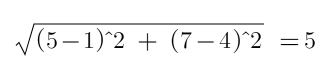Okay, but how can we apply that in machine learning? Imagine to be a bookseller and you want to classify a new book called Ubick of Philip K. Dick with 240 pages which cost 14 euro. As you can see below there are 5 possible classes where to put our new book. 好的，但是我们如何将其应用到机器学习中呢？ 想象成为一个书商，您想对一本名为Philip K. Dick的Ubick的新书进行分类，共有240页，售价14欧元。 如您在下面看到的，有5种可能的类别可用于放置我们的新书。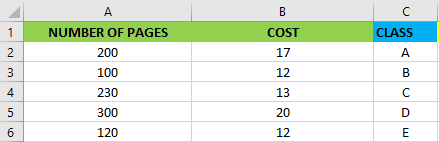image by authorTo know which is the best class for Ubick we can use the euclidian formula in order to compute the distance with each observation in the dataset. 要知道哪个是Ubick的最佳分类，我们可以使用欧几里得公式来计算数据集中每个观测值的距离。
Formula: 式：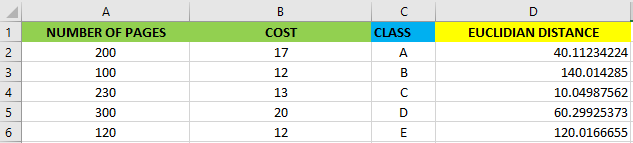image by authorAs you can see above the nearest class for Ubick is class C. 如您所见，Ubick最近的课程是C类 。
Part II: insights that I found to create the model 第二部分：我发现的创建模型的见解
Before to start to speak about the algorithm, that I used to create my model and predict the varieties of wine, let me show you briefly the main insights that I found. 在开始谈论算法之前，我曾用它来创建模型并预测葡萄酒的种类，然后让我简要地向您展示我发现的主要见解。
In the following heatmap, there are correlations between the different features. This is very useful to have a first look at the situation of our dataset and knowing if it is possible to apply a classification algorithm. 在下面的热图中，不同功能之间存在关联。 首先了解一下数据集的情况，并了解是否有可能应用分类算法，这非常有用。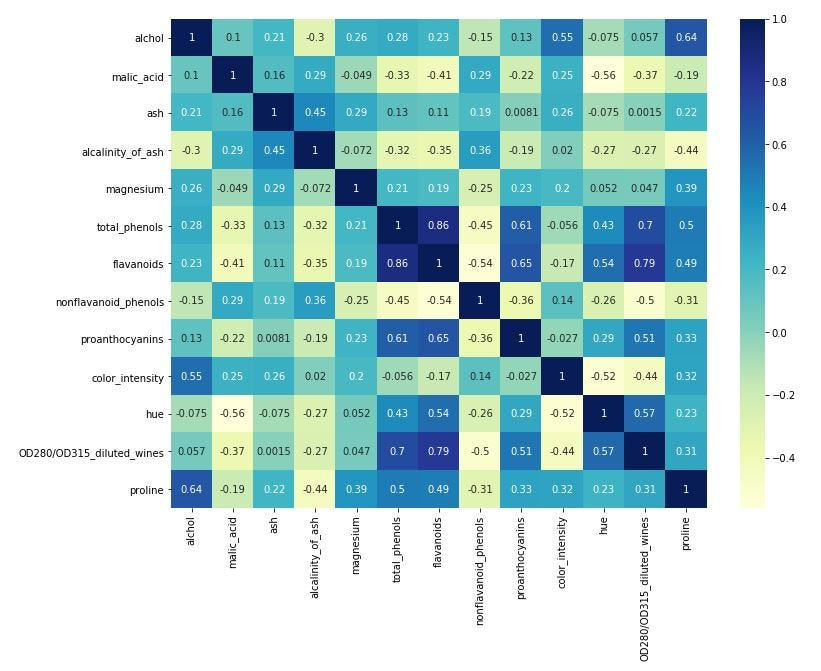image by authorThe heatmap is great for a first look but that is not enough. I’d like also to know if there are some elements whose absolute sum of correlations is low in order to delete them before to train the machine learning model. So, I construct a histogram as you can see below. 该热图乍一看很棒，但这还不够。 我还想知道是否存在某些元素的相关绝对和很低，以便在训练机器学习模型之前将其删除。 因此，如下图所示，我构建了一个直方图。
You can see that there are three elements with low total absolute correlation. The elements are ash, magnesium and the color_intensity. 您会看到三个绝对绝对相关性较低的元素。 元素是灰，镁和color_intensity。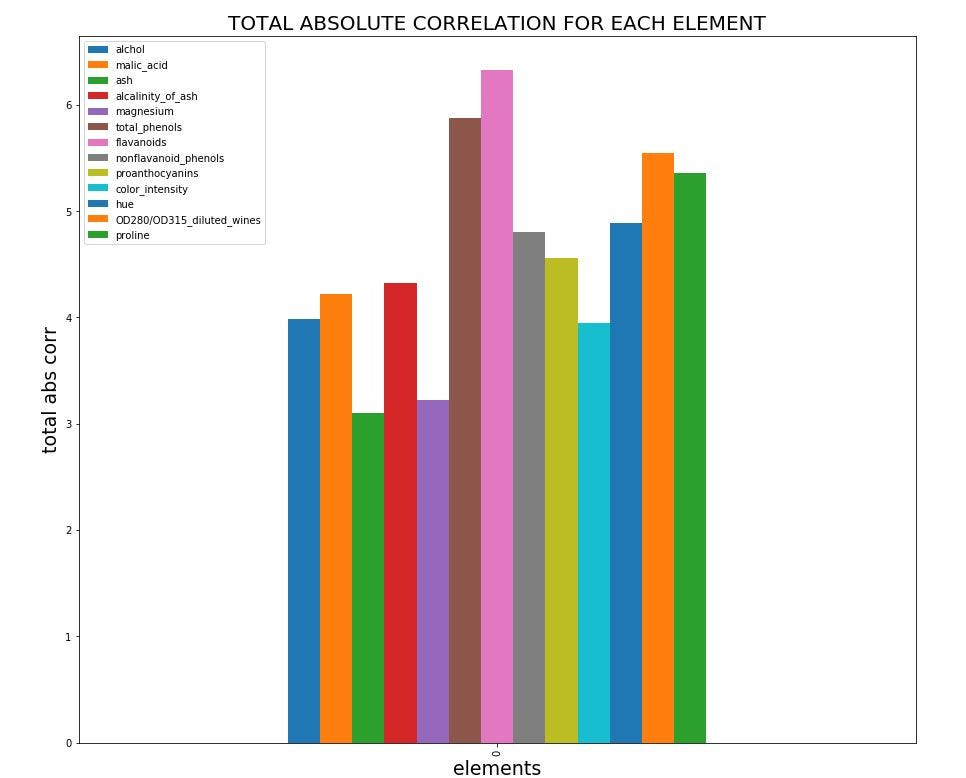image by authorThanks to these observations now we are sure that there is the possibility to apply a KNN algorithm to create a predictive model. 现在，由于这些观察，我们确信可以应用KNN算法创建预测模型。
Part III: use scikit-learn to make predictions 第三部分：使用scikit-learn进行预测
In this part, we will see how to prepare the model and evaluate it thanks to scikit-learn. 在这一部分中，我们将借助scikit-learn了解如何准备模型并进行评估。
Below you can observe that I split the model into two parts: 80% for training and 20% for testing. I chose this proportion because the data set is not big. 在下面，您可以看到我将模型分为两个部分：80％用于训练，20％用于测试。 我选择此比例是因为数据集不大。
# split data to train and test
y = df['class']
X = input_data.drop(columns=['ash','magnesium', 'color_intensity'])

X_train, X_test, y_train, y_test = train_test_split(X, y, test_size = 0.20, random_state=0)

# to be sure that the data was split rightly (80% for train data and 20% for test data)

print("X_train shape: {}".format(X_train.shape))
print("y_train shape: {}".format(y_train.shape))

print("X_test shape: {}".format(X_test.shape))
print("y_test shape: {}".format(y_test.shape))out: 出：
X_train shape: (141, 10)y_train shape: (141,)X_test shape: (36, 10)y_test shape: (36,)You have to know that all machine learning models in scikit-learn are implemented in their own classes. For example, the k-nearest neighbors classification algorithm is implemented in the KNeighborsClassifier class. 您必须知道scikit-learn中的所有机器学习模型都是在各自的类中实现的。 例如，在KNeighborsClassifier类中实现了k最近邻居分类算法。
The first step is to instantiate the class into an object that I called cli as you can see below. The object contains the algorithm that I will use to build the model from the training data and make predictions on new data points. It contains also the information that the algorithm has extracted from the training data. 第一步是将类实例化为一个我称为cli的对象，如下所示。 该对象包含用于从训练数据构建模型并对新数据点进行预测的算法。 它还包含算法已从训练数据中提取的信息。
Finally, to build the model on the training set, we call the fit method of the cli object. 最后，要在训练集上构建模型，我们调用cli对象的fit方法 。
from sklearn.neighbors import KNeighborsClassifier

cli = KNeighborsClassifier(n_neighbors=1)
cli.fit(X_train, y_train)out: 出：
KNeighborsClassifier(algorithm='auto', leaf_size=30, metric='minkowski',metric_params=None, n_jobs=None, n_neighbors=1, p=2,weights='uniform')In the output of the fit method, you can see the parameters used in creating the model. 在fit方法的输出中，您可以看到用于创建模型的参数。
Now, it is time to evaluate the model. Below, the first output shows us that the model predict the 89% of the test data. Instead the second output give us a complete overview of the accuracy for each class. 现在，该评估模型了。 下面的第一个输出向我们展示了该模型预测了89％的测试数据。 相反，第二个输出为我们提供了每个类别的准确性的完整概述。
y_pred = cli.predict(X_test)
print("Test set score: {:.2f}".format(cli.score(X_test, y_test)))

# below the values of the model
from sklearn.metrics import classification_report
print("Final result of the model \n {}".format(classification_report(y_test, y_pred)))out: 出：
Test set score: 0.89out: 出：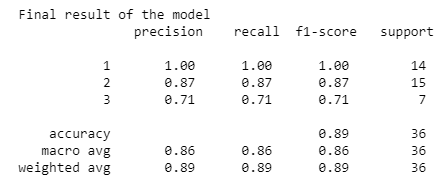Conclusion 结论
I think that the best way to learn something is by practising. So in my case, I download the dataset from Kaggle which is one of the best places where to find a good dataset on which you can apply your machine learning algorithms and learn how they work. 我认为最好的学习方法是练习。 因此，就我而言，我是从Kaggle下载数据集的，这是找到良好数据集的最佳位置之一，您可以在该数据集上应用机器学习算法并了解它们的工作方式。
Thanks for reading this. There are some other ways you can keep in touch with me and follow my work: 感谢您阅读本文。 您可以通过其他方法与我保持联系并关注我的工作：
Subscribe to my newsletter. 订阅我的时事通讯。 You can also get in touch via my Telegram group, Data Science for Beginners. 您也可以通过我的电报小组“ 面向初学者的数据科学”来联系 。 翻译自: https://towardsdatascience.com/machine-learning-observe-how-knn-works-by-predicting-the-varieties-of-italian-wines-a64960bb2daeknn 机器学习
展开全文python 人工智能 java 深度学习
• 本文直接给出sklearn里面KNN 算法的用法。具体实现过程如下： import numpy as np from sklearn import datasets import operator from sklearn import neighbors import sklearn.model_selection as ms ...
本文直接给出sklearn里面KNN 算法的用法。具体实现过程如下：

# -*- coding: utf-8 -*-
import numpy as np
import pandas as pd
from sklearn import datasets
import operator
from sklearn import neighbors
import sklearn.model_selection as ms
import matplotlib.pyplot as plt

totalNum = len(digits.data)
# 选出80%样本作为训练样本，其余20%测试
trainNum = int(0.8 * totalNum)
trainX,testX, trainY,testY = ms.train_test_split(digits.data, digits.target, random_state = 1, train_size = 0.8)

np.shape(digits.data)
np.shape(digits.target)

#用图像来初步认识下特征的长相
X_train = trainX.reshape(len(trainX), 8,8)
X_train = X_train/X_train.max() # 数据归一化
print("After reshaping, the shape of the X_train is:", X_train.shape)
np.shape(X_train)
a = X_train
a.shape
plt.imshow(a, cmap = 'Greys_r')  #画图
#训练模型，并计算不同K的情况下ER的变化情况
ER = []
for n_neighbors  in range(1,16):
clf = neighbors.KNeighborsClassifier(n_neighbors, weights='uniform') #测试不同的K 对最终结果的影响
clf.fit(trainX, trainY) #训练器
Z = clf.predict(testX)  #预测
x = 1- np.mean(Z == testY) #计算错误率
ER.append(x) #将错误率储存在ER 中
pd.DataFrame(ER).plot(title = 'the plot of error rate') #画图显示不同K对模型正确的影响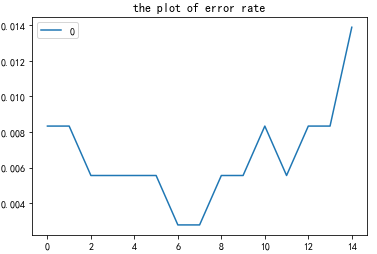通过以上的图形可知，n_neighbors = 7,8 时较为合适， 此时的error rate 为0.002778
# -*- coding: utf-8 -*-
import numpy as np
from sklearn import neighbors, datasets
from sklearn.model_selection import train_test_split
from sklearn.utils.testing import assert_equal

rng = np.random.RandomState(0)
perm = rng.permutation(digits.target.size)
digits.data = digits.data[perm]
digits.target = digits.target[perm]

def test_neighbors_digits():
# Sanity check on the digits dataset
# the 'brute' algorithm has been observed to fail if the input
# dtype is uint8 due to overflow in distance calculations.

X = digits.data.astype('uint8')
Y = digits.target
(n_samples, n_features) = X.shape
train_test_boundary = int(n_samples * 0.8)
train = np.arange(0, train_test_boundary)
test = np.arange(train_test_boundary, n_samples)
(X_train, Y_train, X_test, Y_test) = X[train], Y[train], X[test], Y[test]
clf = neighbors.KNeighborsClassifier(n_neighbors=1, algorithm='brute')
clf_unit8 = clf.fit(X_train, Y_train)
clf_float = clf.fit(X_train.astype(float), Y_train)
score_uint8 = clf_unit8.score(X_test, Y_test)
score_float = clf_float.score(X_test.astype(float), Y_test)
assert_equal(score_uint8, score_float)
pred_y = clf_unit8.predict(X_test)
print("the acurracy rate is :", np.mean(pred_y == Y_test))
test_neighbors_digits()

以下是机器学习实战书中的源代码。

# -*- coding: utf-8 -*-
"""
Created on Mon Sep 17 15:03:26 2018
"""
from numpy import *
import operator

def classify0(inX, dataSet, labels, k):
dataSetSize = dataSet.shape
diffMat = tile(inX, (dataSetSize,1)) - dataSet
sqDiffMat = diffMat**2
sqDistances = sqDiffMat.sum(axis=1)
distances = sqDistances**0.5
sortedDistIndicies = distances.argsort()
classCount={}
for i in range(k):
voteIlabel = labels[sortedDistIndicies[i]]
classCount[voteIlabel] = classCount.get(voteIlabel,0) + 1
sortedClassCount = sorted(classCount.items(), key=operator.itemgetter(1), reverse=True)
return int(sortedClassCount)

def createDataSet():
group = array([[1.0,1.1],[1.0,1.0],[0,0],[0,0.1]])
labels = ['A','A','B','B']
return group, labels

def file2matrix(filename):
fr = open(filename)
numberOfLines = len(fr.readlines())         #get the number of lines in the file
returnMat = zeros((numberOfLines,3))        #prepare matrix to return
classLabelVector = []                       #prepare labels return
fr = open(filename)
index = 0
line = line.strip()
listFromLine = line.split('\t')
returnMat[index,:] = list(map(float,listFromLine[0:3]))
classLabelVector.append(int(listFromLine[-1]))
index += 1
return returnMat,classLabelVector

#normalize
def autoNorm(dataSet):
minVals = dataSet.min(0)
maxVals = dataSet.max(0)
ranges = maxVals - minVals
normDataSet = zeros(shape(dataSet))
m = dataSet.shape
normDataSet = dataSet - tile(minVals, (m,1))
normDataSet = normDataSet/tile(ranges, (m,1))   #element wise divide
return normDataSet, ranges, minVals

#autoNorm(datingDataMat)

def datingClassTest():
hoRatio = 0.50      #hold out 10%
datingDataMat,datingLabels = file2matrix(path+'/datingTestSet2.txt')       #load data setfrom file
normMat, ranges, minVals = autoNorm(datingDataMat)
m = normMat.shape
numTestVecs = int(m*hoRatio)
errorCount = 0.0
for i in range(numTestVecs):
classifierResult = classify0(normMat[i,:],normMat[numTestVecs:m,:],datingLabels[numTestVecs:m],3)
print ("the classifier came back with: %d, the real answer is: %d" % (classifierResult, datingLabels[i]))
if (classifierResult != datingLabels[i]): errorCount += 1.0
print ("the total error rate is: %f" % (errorCount/float(numTestVecs)))
print (errorCount)

#datingClassTest()

###########################################################deal with digit
from os import listdir

#将数据转化成1*1024的举证
def img2vector(filename):
returnVect = zeros((1,1024))
fr = open(filename)
for i in range(32):
for j in range(32):
returnVect[0,32*i+j] = int(lineStr[j])
return returnVect

def handwritingClassTest():
hwLabels = []
trainingFileList = listdir(pat + '/trainingDigits')           #load the training set
m = len(trainingFileList)
trainingMat = zeros((m,1024))
for i in range(m):
fileNameStr = trainingFileList[i]
fileStr = fileNameStr.split('.')     #take off .txt
classNumStr = int(fileStr.split('_'))
hwLabels.append(classNumStr)
trainingMat[i,:] = img2vector(pat +'/trainingDigits/%s' % fileNameStr)
testFileList = listdir(pat +'/testDigits')        #iterate through the test set
errorCount = 0.0
mTest = len(testFileList)
for i in range(mTest):
fileNameStr = testFileList[i]
fileStr = fileNameStr.split('.')     #take off .txt
classNumStr = int(fileStr.split('_'))
vectorUnderTest = img2vector(pat +'/testDigits/%s' % fileNameStr)
classifierResult = classify0(vectorUnderTest, trainingMat, hwLabels, 3)
print ("the classifier came back with: %d, the real answer is: %d" % (classifierResult, classNumStr))
if (classifierResult != classNumStr): errorCount += 1.0
print ("\nthe total number of errors is: %d" % errorCount)
print ("\nthe total error rate is: %f" % (errorCount/float(mTest)))
return classifierResult, classNumStr, trainingMat

#以下为测试代码：
if __main__ == '__name__':
datingDataMat, datingLabels = file2matrix(path + '/datingTestSet2.txt')
import matplotlib.pyplot as plt
import seaborn as sns
import pandas as pd
d1 = pd.DataFrame(data = datingDataMat, columns = ['km', 'GameTime', 'IceCream'])
d2 = pd.DataFrame(datingLabels, columns = ['label'])
df = pd.concat([d1, d2], axis = 1)
df.info()
g = sns.FacetGrid(data = df, hue = 'label', size = 6, palette='Set2')
ax = sns.countplot(x = 'label', data = df, palette= 'Set3') #数据均匀分布
ax = sns.boxplot(y = 'GameTime', x = 'label', data = df, palette= 'Set3')
ax = sns.boxplot(y = 'IceCream', x = 'label', data = df, palette= 'Set3')
ax = sns.boxplot(y = 'km', x = 'label', data = df, palette= 'Set3')
g = sns.FacetGrid(data= df, hue = 'label', size = 6, palette='Set3')
handwritingClassTest()
zero = trainingMat[8,:]
img_0 = zero.reshape(32,32)
plt.imshow(img_0)



展开全文• 参考博文：...数据格式：user item rating timestamp安装库： 在安装surprise库的时候如果用python3.X的时候会提示需要visio c++ 2014，但是笔者环境明明有visio c++2014和2015，具体好像还需要一些其...
参考博文：https://blog.csdn.net/jsond/article/details/73042866本文仅为记录尝试的时候遇到的坑。数据格式：user item rating timestamp安装库：    在安装surprise库的时候如果用python3.X的时候会提示需要visio c++ 2014，但是笔者环境明明有visio c++2014和2015，具体好像还需要一些其他配置，并没有去深究，后经搜索用python2.7可以直接安装使用：在安装之前首先要确认已经安装了numpy库。pip install scikit-surprise源码如下：# -*- coding:utf-8 -*-
from __future__ import (absolute_import, division, print_function, unicode_literals)
import os
import io
from surprise import KNNBaseline
from surprise import Dataset

import logging

logging.basicConfig(level=logging.INFO,
format='%(asctime)s %(filename)s[line:%(lineno)d] %(levelname)s %(message)s',
datefmt='%a, %d %b %Y %H:%M:%S')

# 训练推荐模型 步骤:1
def getSimModle():
# 默认载入movielens数据集
trainset = data.build_full_trainset()
#使用pearson_baseline方式计算相似度  False以item为基准计算相似度 本例为电影之间的相似度
sim_options = {'name': 'pearson_baseline', 'user_based': False}
##使用KNNBaseline算法
algo = KNNBaseline(sim_options=sim_options)
#训练模型
algo.train(trainset)
return algo

# 获取id到name的互相映射  步骤:2
"""
获取电影名到电影id 和 电影id到电影名的映射
"""
file_name = (os.path.expanduser('~') +
'/.surprise_data/ml-100k/ml-100k/u.item')
rid_to_name = {}
name_to_rid = {}
with io.open(file_name, 'r', encoding='ISO-8859-1') as f:
for line in f:
line = line.split('|')
rid_to_name[line] = line
name_to_rid[line] = line
return rid_to_name, name_to_rid

# 基于之前训练的模型 进行相关电影的推荐  步骤：3
def showSimilarMovies(algo, rid_to_name, name_to_rid):
# 获得电影Toy Story (1995)的raw_id
toy_story_raw_id = name_to_rid['Toy Story (1995)']
logging.debug('raw_id=' + toy_story_raw_id)
#把电影的raw_id转换为模型的内部id
toy_story_inner_id = algo.trainset.to_inner_iid(toy_story_raw_id)
logging.debug('inner_id=' + str(toy_story_inner_id))
#通过模型获取推荐电影 这里设置的是10部
toy_story_neighbors = algo.get_neighbors(toy_story_inner_id, 10)
logging.debug('neighbors_ids=' + str(toy_story_neighbors))
#模型内部id转换为实际电影id
neighbors_raw_ids = [algo.trainset.to_raw_iid(inner_id) for inner_id in toy_story_neighbors]
#通过电影id列表 或得电影推荐列表
neighbors_movies = [rid_to_name[raw_id] for raw_id in neighbors_raw_ids]
print('The 10 nearest neighbors of Toy Story are:')
for movie in neighbors_movies:
print(movie)

if __name__ == '__main__':
# 获取id到name的互相映射

# 训练推荐模型
algo = getSimModle()

##显示相关电影
showSimilarMovies(algo, rid_to_name, name_to_rid)数据集路径问题：    1、第一次运行的时候总是会在read_item_names()函数中第一句提醒找不到ml-100k的数据集文件，后经查阅os.path.expanduser(path)  的作用是：把path中包含“~”和“~user”转换成用户目录。后自己去单独下载了ml-100k数据集，并放在同级目录下，然后将单引号中路径换为‘/ml-100k/u.item’，还是找不到。再把os.path.expanduser('~')去掉，不通过此方式，后发现不抱错，应该是找到了对应文件。    2、接下来在getSimModel()函数中，提醒需要下载ml-100k数据集，这里好像是直接使用surprise库中Dataset的数据集，按照提示下载即可，如果太慢的话，用蓝灯开VPN进行下载。运行：    之后虽然会有报警告，但是已经可以正常运行了：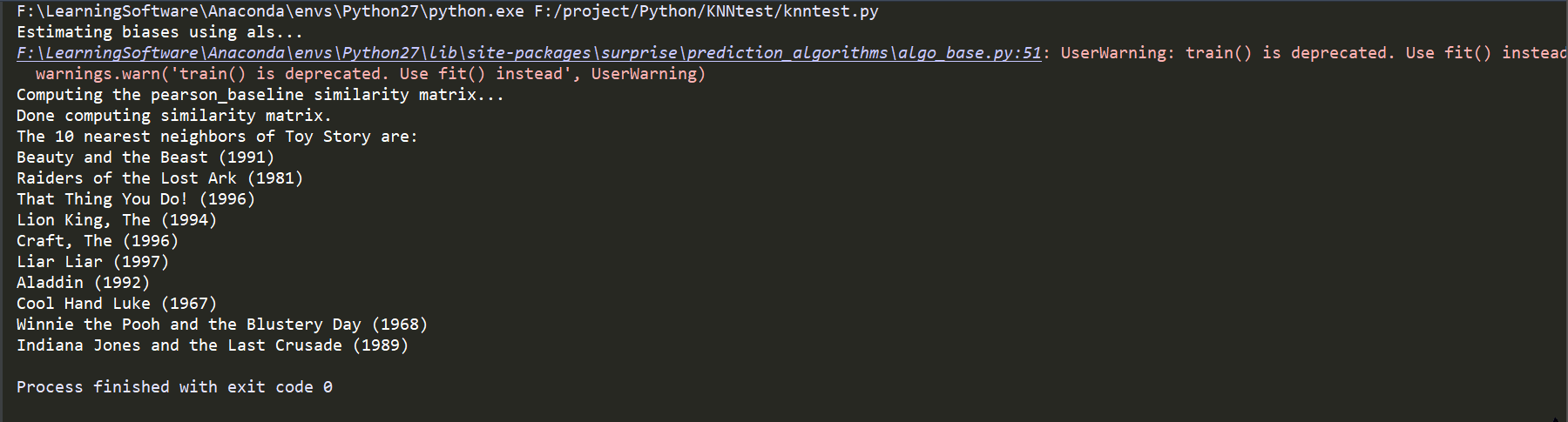此处输出就是Toy Story （1995）最相近的10部电影。尝试将参数换为Beauty and the Beast(1991)，输出结果如下：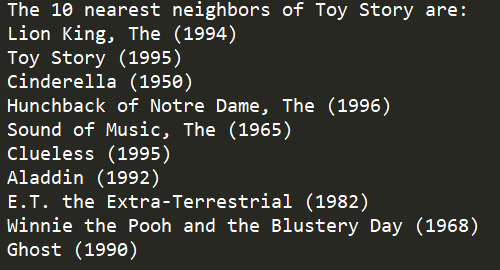同样Toy Story(1995)也在其中。数据：/ml-100k/u.item内容如下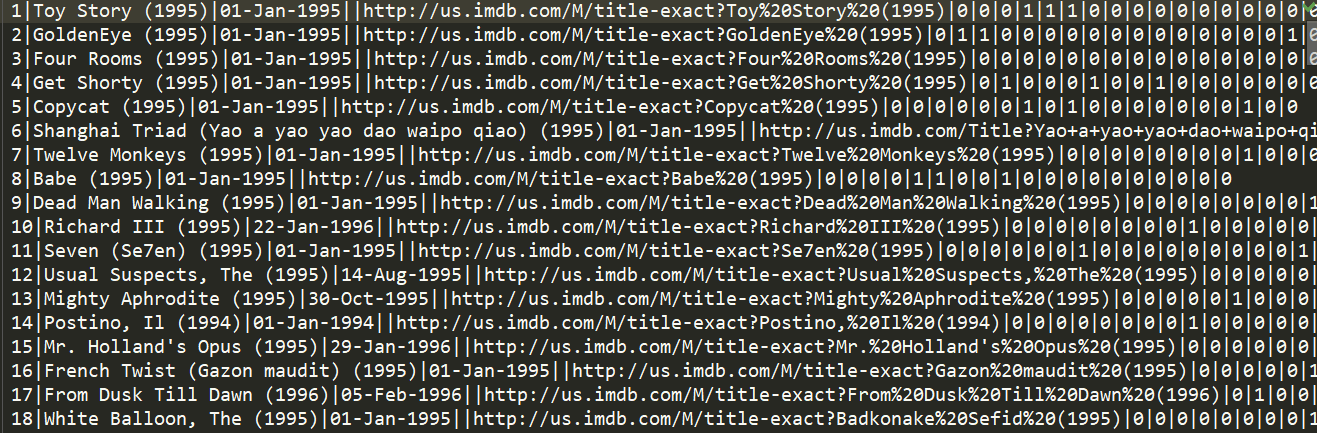尾部的参数就是根据电影以及影评人的评分所构造的矩阵。
展开全文• KNN算法是监督学习分类方法。何为监督学习？我们用来训练的数据集应当包括数据特征和标签两个部分，通过训练建立数据特征和标签之间关系的算法模型，这样的话，将测试数据集套用算法模型，可以得到测试数据的标签。...
• ## 机器学习之KNN最邻近分类算法

万次阅读 多人点赞 2018-09-15 13:13:33
KNN算法简介 KNN（K-Nearest Neighbor）最邻近分类算法是数据挖掘分类（classification）技术中最简单的算法之一，其指导思想是”近朱者赤，近墨者黑“，即由你的邻居来推断出你的类别。 KNN最邻近分类算法的...最邻近算法 python
• 一、KNN算法简介： 用一句通俗易懂的话来形容KNN算法，便是：“近朱者赤，近墨者黑”。为什么这么说呢？看看它的的算法原理吧。 算法原理：计算测试样本与每个训练样本的距离（距离计算方法见下文），取前k个距离...
• kNN机器学习里面最简单的一个入门算法。 这里面主要提供两个例子，以及对应的样本集 目录： 一 算法流程 二 约会推荐系统 三 手写数字识别系统 一 算法流程： 1.1 输入： 样本示例集合 测试...
• 机器学习算法——KNN KNN算法和KD-Tree 思维导图
• KNN算法的机器学习基础 https://mp.weixin.qq.com/s/985Ym3LjFLdkmqbytIqpJQ 本文原标题 ： Machine Learning Basics with the K-Nearest Neighbors Algorithm 翻译 | 小哥哥、江舟 校对 | 吕鑫灿 整理 | 志豪 ...
• 11.27机器学习实战之KNN初探 摘抄的别人的链接 这个文章写的非常详细~~~ (https://blog.csdn.net/c406495762/article/details/75172850) K-近邻法 k近邻法(k-nearest neighbor, k-NN)是1967年由Cover T和Hart P提出...
• 这里写自定义目录标题kNN思想新的改变功能快捷键合理的创建标题，有助于目录的生成如何改变文本的样式插入链接与图片如何插入一段漂亮的代码片生成一个适合你的列表创建一个表格设定内容居中、居左、居右SmartyPants...笔记
• # -*- coding:utf-8 -*- import numpy as np class KNN(object): def __init__(self, k): self.k = k def fit(self, x, y): self.x_train = np.asarray(x) self.y_train = np.as...
• KNN算法的学习 KNN的英文叫K-Nearest Neighbor，比较简单 一、简单的例子 首先我们先从一个简单的例子入手，来体会一下KNN算法。 假设，我们想对电影的类型进行分类，统计了电影中打斗的次数、接吻的次数，当然还有...
• 机器学习-KNN算法学习（一） 目标：掌握KNN算法的基本概念、优缺点以及代码实现。 文章目录机器学习-KNN算法学习（一）一、KNN算法简介1、KNN(k-Nearest Neighbours)概念2、KNN算法优缺点3、KNN算法一般流程二、...python
• 算法描述步骤 为了判断未知实例的类别，以所有已知类别的实例作为参照 选择参数K 计算未知实例与所有已知实例的距离 选择最近K个已知实例 ...细节关于K关于距离的衡量方法:Euclidean Distance 定义 其他距离衡量：余弦...
• KNN是什么？ KNN的英文是k-NearestNeighbor(K最近邻),是一种邻近算法。 K是什么？ KNN通过依据k个对象中占优的类别进行决策.它的主要思想是看这个数据距离最近的 K 个节点中,这些节点哪个类占最多 那怎么选取范围呢...
• 机器学习算法 机器学习的任务可分为回归与分类 对于分类算法，通常我们输入大量已分类数据作为算法的训练集，训练集为训练样本的集合 每个训练样本包含特征（也称属性）以及目标变量，在分类算法中，我们目标变量...Python
• 刚刚开始在一个视频上学习机器学习，不懂的还是很多，这也算作是学习机器学习的笔记吧 KNN算法，K nearest neighbor 最近的K个邻居，了解一个算法，先从了解一个问题开始，现在问题如下，有很多的数字图片，每个...
• 包含基于Python实现的kNN代码，可在Pycharm中直接运行，包含约会网站的配对改进程序以及手写体识别系统
• K最近邻(k-Nearest Neighbor，以下简称KNN)分类算法，是一个理论上比较成熟的方法，也是最简单的机器学习算法之一。该方法的思路是：如果一个样本在特征空间中的k个最相似(即特征空间中最邻近)的样本中的大多数属于...人工智能
• 下面是《机器学习实战》中的KNN分类算法的笔记。自我觉得学了一段时间的机器学习KNN可以说是公式推导最简单，最容易理解的一个算法了。资源已经上传，如果有需要请到如下链接下载:...
• KNN简介 邻近算法，或者说K最近邻(kNN，k-NearestNeighbor)分类算法是数据挖掘分类技术中最简单的方法之一。所谓K最近邻，就是k个最近的邻居的意思，说的是每个样本都可以用它最接近的k个邻居来代表。 kNN算法的...python
• 今天介绍机器学习中比较常见的一种分类算法，K-NN，NN 就是 Nearest Neighbors， 也就是最近邻的意思，这是一种有监督的分类算法，给定一个 test sample, 计算这个 test sample 与 training set 里每个 training ...python
• KNN（最邻近规则分类K-Nearest-Neighibor)KNN算法 1. 综述 1.1 Cover和Hart在1968年提出了最初的邻近算法 1.2 分类(classification)算法 1.3 输入基于实例的学习(instance-based learning), 懒惰学习(lazy ...
• 1.过程:计算测试样本与训练样本之间的距离，这里的距离有欧式距离，曼哈顿距离，拉普拉斯距离等。按照距离进行排序选择其中最近的k个值，这里k值的选择用到交叉验证的方法，交叉验证包括s折，随机，留一根据分类决策...
• KNN实战1、KNN算法的一般流程1、搜集数据：可以使用任何方法2、准备数据：距离计算所需要的数值，最好是结构化的数据格式3、分析数据：可以使用任何方法4、训练算法：此...学习《机器学习实战》 1、KNN算法的一般......